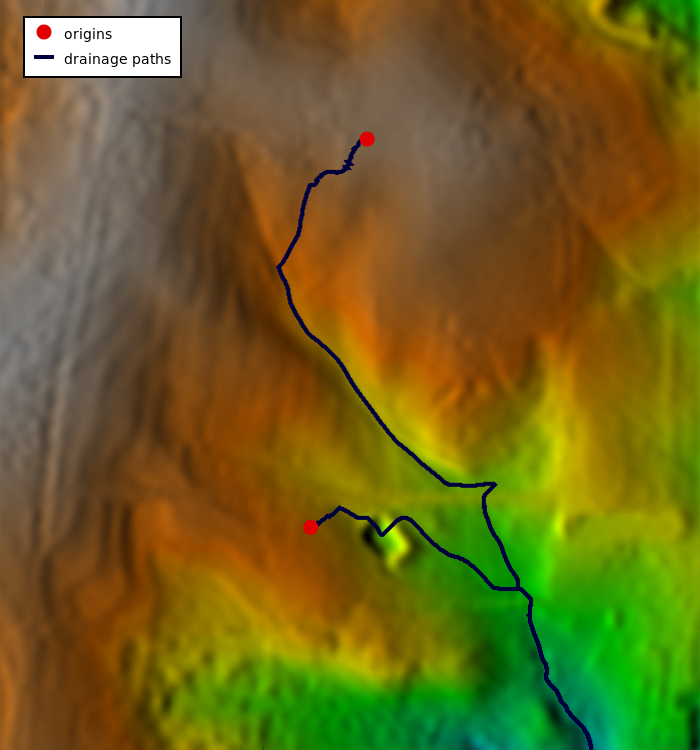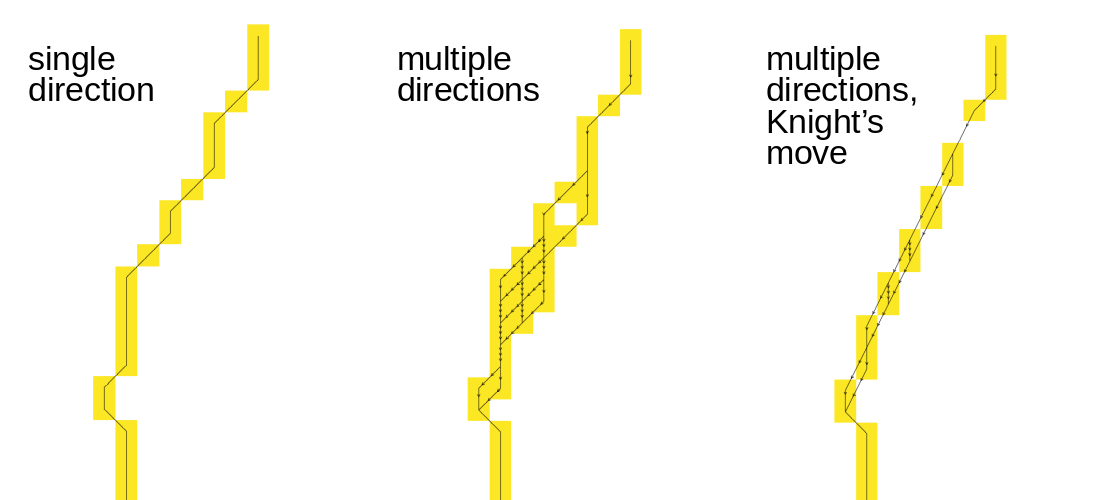## NAME

r.path - Traces paths from starting points following input directions.

## KEYWORDS

raster, hydrology, cost surface

## SYNOPSIS

r.path
r.path --help
r.path [-can] input=name format=string [values=name] [raster_path=name] [vector_path=name] [start_coordinates=east,north[,east,north,...]] [start_points=name[,name,...]] [--overwrite] [--help] [--verbose] [--quiet] [--ui]

### Flags:

-c
Copy input cell values on output
-a
Accumulate input values along the path
-n
Count cell numbers along the path
--overwrite
Allow output files to overwrite existing files
--help
Print usage summary
--verbose
Verbose module output
--quiet
Quiet module output
--ui
Force launching GUI dialog

### Parameters:

input=name [required]
Name of input direction
Direction in degrees CCW from east, or bitmask encoded
format=string [required]
Format of the input direction map
Default: auto
auto: auto-detect direction format
degree: degrees CCW from East
45degree: degrees CCW from East divided by 45 (e.g. r.watershed directions)
values=name
Name of input raster values to be used for output
Name of input raster map
raster_path=name
Name for output raster path map
Name for output raster map
vector_path=name
Name for output vector path map
Name for output vector map
start_coordinates=east,north[,east,north,...]
Coordinates of starting point(s) (E,N)
start_points=name[,name,...]
Name of starting vector points map(s)
Or data source(s) for direct OGR access

## DESCRIPTION

r.path traces a path from starting points following input directions. Such a movement direction map can be generated with r.walk, r.cost, r.slope.aspect, r.watershed, or r.fill.dir, provided that the direction is in degrees, measured counterclockwise from east.

Alternatively, bitmask-encoded directions can be provided where each bit position corresponds to a specific neighbour. A path will continue to all neighbours with their bit set. This means a path can split and merge. Such bitmasked directions can be created with the -b flag of r.cost and r.walk.

```Direction encoding for neighbors of x

135  90  45          7 8 1
180  x  360          6 x 2
225 270 315          5 4 3

degrees           bit positions
CCW from East
```
A path stops when the direction is zero or negative, indicating a stop point or outlet.

The output raster map will show one or more least-cost paths between each user-provided location(s) and the target points (direction ≤ 0). By default, the output will be an integer CELL map with the id of the start points along the least cost path, and null cells elsewhere.

With the -c (copy) flag, the values raster map cell values are copied verbatim along the path. With the -a (accumulate) flag, the accumulated cell value from the starting point up to the current cell is written on output. With either the -c or the -a flags, the raster_path map is created with the same cell type as the values raster map (integer, float or double). With the -n (number) flag, the cells are numbered consecutively from the starting point to the final point. The -c, -a, and -n flags are mutually incompatible.

The start_coordinates parameter consists of map E and N grid coordinates of a starting point. Each x,y pair is the easting and northing (respectively) of a starting point from which a path will be traced following input directions. The start_points parameter can take multiple vector maps containing additional starting points.

## NOTES

The directions are recorded as degrees CCW from East, the Knight's move of r.cost and r.walk is considered:
```       112.5     67.5
157.5  135   90  45   22.5
180   x   0
202.5  225  270  315  337.5
247.5     292.5
```
i.e. a cell with the value 135 means the next cell is to the North-West, and a cell with the value 157.5 means that the next cell is to the West-North-West.

## EXAMPLES

### Hydrological path

We are using the full North Carolina sample dataset. First we create the two points from a text file using v.in.ascii module (here the text file is CSV and we are using unix here-file syntax with EOF, in GUI just enter the values directly for the parameter input):
```v.in.ascii input=- output=start format=point separator=comma <<EOF
638667.15686275,220610.29411765
638610.78431373,220223.03921569
EOF
```
We need to supply a direction raster map to the r.path module. To get these directions, we use the r.watershed module:
```r.watershed elevation=elev_lid792_1m accumulation=accum drainage=drain_dir
```
The directions are categorical and we convert them to degrees using raster algebra:
```r.mapcalc "drain_deg = if(drain_dir != 0, 45. * abs(drain_dir), null())"
```
Now we are ready to extract the drainage paths starting at the two points.
```r.path input=drain_deg raster_path=drain_path vector_path=drain_path start_points=start
```
Before we visualize the result, we set a color table for the elevation we are using and create a shaded relief map:
```r.colors map=elev_lid792_1m color=elevation
r.relief input=elev_lid792_1m output=relief
```
We visualize the input and output data:
```d.shade shade=relief color=elev_lid792_1m
d.vect map=drain_path color=0:0:61 width=4 legend_label="drainage paths"
d.vect map=start color=none fill_color=224:0:0 icon=basic/circle size=15 legend_label=origins
d.legend.vect -b
```Figure: Drainage paths from two points where directions from r.watershed were used

### Least-cost path

We compute bitmask encoded movement directions using r.walk:
```g.region swwake_30m -p

# create friction map based on land cover
r.recode input=landclass96 output=friction rules=- << EOF
1:3:0.1:0.1
4:5:10.:10.
6:6:1000.0:1000.0
7:7:0.3:0.3
EOF

# without Knight's move
r.walk -b elevation=elev_ned_30m friction=friction output=walkcost \
outdir=walkdir start_coordinates=635576,216485

r.path input=walkdir start_coordinates=640206,222795 \
raster_path=walkpath vector_path=walkpath

# with Knight's move
r.walk -b -k elevation=elev_ned_30m friction=friction output=walkcost_k \
outdir=walkdir_k start_coordinates=635576,216485

r.path input=walkdir_k start_coordinates=640206,222795 \
raster_path=walkpath_k vector_path=walkpath_k

# without Knight's move and without bitmask encoding (single direction)
r.walk elevation=elev_ned_30m friction=friction output=walkcost_s \
outdir=walkdir_s start_coordinates=635576,216485

r.path input=walkdir_s start_coordinates=640206,222795 \
raster_path=walkpath_s vector_path=walkpath_s
```
The extracted least-cost path splits and merges on the way from the start point to the stop point (start point for r.walk). Note the gaps in the raster path when using the Knight's move.Figure: Comparison of shortest paths using single directions and multiple bitmask encoded directions without and with Knight's move

g.region, r.basins.fill, r.cost, r.fill.dir, r.mapcalc, r.recode, r.terraflow, r.walk, r.watershed

## AUTHOR

Markus Metz
Multiple path directions sponsored by mundialis

## SOURCE CODE

Available at: r.path source code (history)

Latest change: Monday Jan 30 09:36:46 2023 in commit: 0fb05e493f4ef73f4a721b019bffc357be2ec8d6

© 2003-2023 GRASS Development Team, GRASS GIS 8.3.1dev Reference Manual